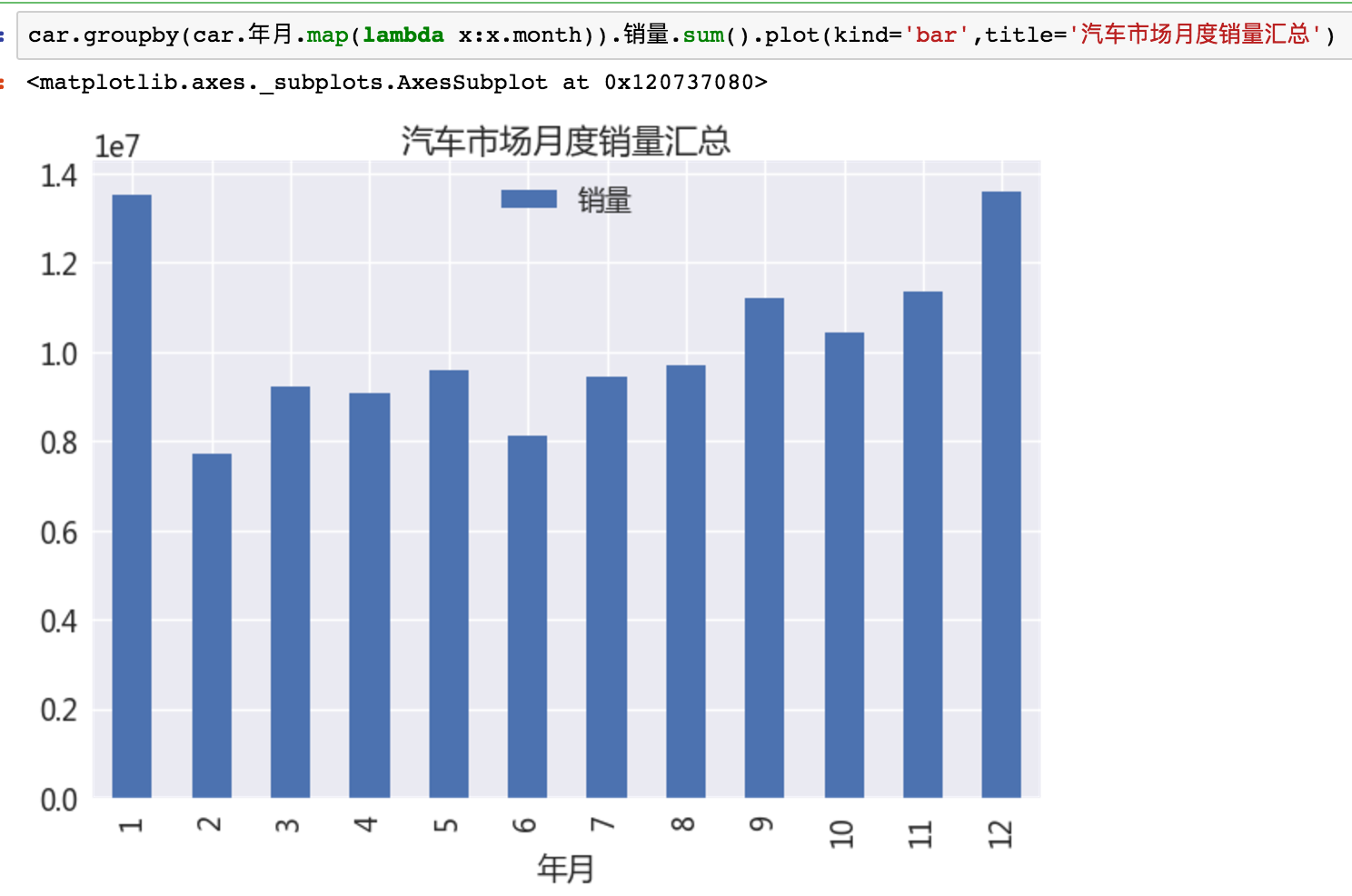# Python数据分析之路（一）查询和统计

## 0. 如何入门数据分析

1. 查询与统计
1. 可视化和高级用法
1. 分类和预测（估计会分为几篇文章）

## 1. 找到好的问题

1. 我们知道自己知道的（房价在涨）
2. 知道自己不知道的（可度量的信息，如房价平均涨幅）
3. 不知道自己知道的（如证明摇号系统漏洞）
4. 不知道自己不知道的（最有价值，蕴含着最大的机会）

• 我是否找到了一个值得解决的问题？
• 我是否提出了一个足够好的解决方案
• 我真的想去解决这个问题吗？## 2. 查询和过滤

DataFrame是pandas的核心数据结构，可以理解为Excel里的二维矩阵，它更高级，能表达3维或更高维的数据，支持多索引。在内存中存取，效率极高，绝大多数操作都和DataFrame相关。维度为2的DataFrame，行(column)和列(row)的axis分别为0和1。可以针对某些列做索引(index),高维DataFrame是相当少见的。

### 2.1 查询

[] 列值索引 df['房价'] ,df[['房价','车价']]
loc 行值索引 df.loc, df['2013':'2015']
iloc 行号索引 df.iloc, df.iloc[2:10]
ix 行索引 df.ix, df.ix[2:10]

weather.index= weather[u'日期'].map(lambda x:Timestamp(x))

Pandas的索引功能非常强大，补充如下：

1. loc也能支持先行后列的查询：df.loc['20130101':'20130103' , ['A','B']],类似的如iloc
1. 个人感觉ix的有些冗余，和ixiloc类似

### 2.2 过滤

• 针对特定数据做分析，
• 过滤异常值。

df=df[!data.col.isnull()]

db[db.city.str.contains(u'市')]

qq['id'].unique()

isin能判断单元格中的值是否在给定的数组内，若希望对多个列做过滤，Pandas提供了现成的方法df.filter，还支持正则。还能进行逻辑操作，实现更复杂的需求。

### 2.3 遍历

map 一列的cell cell 最为常见
apply 列/行 列或行的Series axis:不填写cell,1:行,2:列
applymap cell cell element-wise最为灵活
iterrows 行遍历 提供行号 见备注
iteritems 列遍历 提供列名 见备注
itertuples 行遍历 提供index 见备注

map, apply,applymap是只返回单元格或行列本身的，参数都是lambda,本节假设读者对python的lambda表达式有足够的了解。

for i,row in data.iterrows():
pass


### 2.4 求值和合并

table['面积']= np.round(table['总价']*10000/table['单价'])


numpy提供了绝大多数常见的函数算子，能满足大部分需求。下面是合并：

• 横向合并（需保证行数一致）- 横向合并 df = pd.concat([data_train, dummies_Cabin])
• 删除列 df.drop(['Pclass', 'Name', 'Sex')]
• 纵向合并（join操作）
data.merge(right=prop_rates, how='inner',left_on='Property_Area',right_index=True, sort=False)


## 3. 分组，排序，聚合

### 3.1 分组

df2.groupby(df2.日期.map(lambda x:x.year))

Pandas也能支持传递多个列的数组，除了切片以外，能在索引上使用的基本都能在group,sort上使用，一致性的API上手非常容易。

db[db.city==u'北京'][u'平均值'].resample('12M').aqi.mean()

### 3.2 排序

Pandas的排序非常之快，大部分操作都能在瞬间完成。排序分为两类：

#### 对分组后数据排序

groupby之后的数据，和一般的DataFrame不同，而像个字典(dict)。对键排序，需使用sort_index，值排序，需使用sort_values。

### 3.3 聚合

df2.groupby(df2.日期.map(lambda x:x.year)).size()

car.groupby(car.年月.map(lambda x:x.month)).销量.sum().plot(kind='bar',title='汽车市场月度销量汇总')Pandas支持直接将聚合结果绘图输出（虽然丑但是方便啊），下一节我们将详细介绍它的使用细节。

df[(df.省=='广西') & (df.车型分类=='MPV') & (df.品牌=='东风')].groupby('车型').size().sort_values(ascending=False)

### 3.4 数据透视表

http://python.jobbole.com/81212/

pd.pivot_table(df,index=["Name","Rep","Manager"],values=["Price"],aggfunc=[np.sum,len],fill_value=0)pivot之后，生成的DataFrame是multiindex的，处理起来稍显繁琐，用xs可将某个子index的数据“提升”出来，例如:

df_pivot.xs(('12AM新坐标',2011))

## 4. 总结

Pandas本身异常强大，功能非常繁杂，笔者仅仅掌握了其中非常小的一部分。但是对于一般的需求都能通过简单的操作组合出来。API的一致性非常重要，Pandas（包括numpy等）都继承了Python的优良特性，因此只要能举一反三，就能进步神速。

posted @ 2017-07-10 08:33  FerventDesert  阅读(15169)  评论(1编辑  收藏  举报